# Build Technical Indicators In Python

Technical Indicator is essentially a mathematical representation based on data sets such as price (high, low, open, close, etc.) or volume of security to forecast price trends.

There are several kinds of technical indicators that are used to analyse and detect the direction of movement of the price (for momentum trading, mean reversion trading etc). Traders use them to study the short-term price movement since they do not prove very useful for long-term investors. They are employed primarily to predict future price levels.

In the following post, I will highlight six technical indicators that are popularly used in the markets to study the price movement.

Technical Indicators do not follow a general pattern, meaning, they behave differently with every security. What can be a good indicator for a particular security, might not hold the case for the other. Thus, using a technical indicator requires jurisprudence coupled with good experience.

As these analyses can be done in python, a snippet of code is also inserted along with the description of the indicators. Sample charts with examples are also appended for clarity.## Commodity Channel Index (CCI)

The commodity channel index (CCI) is an oscillator which was originally introduced by Donald Lambert in 1980. CCI can be used to identify cyclical turns across asset classes, be it commodities, indices, stocks, or ETFs. CCI is also used by traders to identify overbought/oversold levels for securities.

### Estimation

The CCI looks at the relationship between price and a moving average. Steps involved in the estimation of CCI include:

• Computing the typical price for security. The typical price is obtained by the averaging the high, low and the close price for the day.
• Finding the moving average for the chosen number of days based on the typical price.
• Computing the standard deviation for the same period as that used for the MA.

The formula for CCI is given by:

CCI = (Typical price – MA of Typical price) / (0.015 * Standard deviation of Typical price)

The index is scaled by an inverse factor of 0.015 to provide for more readable numbers.

### Analysis

CCI can be used to determine overbought and oversold levels. Readings above +100 can imply an overbought condition, while readings below −100 can imply an oversold condition. However, one should be careful because security can continue moving higher after the CCI indicator becomes overbought. Likewise, securities can continue moving lower after the indicator becomes oversold.

Whenever the security is in overbought/oversold levels as indicated by the CCI, there is a good chance that the price will see corrections. Hence a trader can use such overbought/oversold levels to enter in short/long positions.

Traders can also look for divergence signals to take suitable positions using CCI. A bullish divergence occurs when the underlying security makes a lower low and the CCI forms a higher low, which shows less downside momentum. Similarly, a bearish divergence is formed when the security records a higher high and the CCI forms a lower high, which shows less upside momentum.

### Python code for computing the Commodity Channel Index

In the code below we use the Series, rollingmean, rollingstd, and the join functions to compute the Commodity Channel Index. The Series function is used to form a series which is a one-dimensional array-like object containing an array of data. The rollingmean function takes a time series or a data frame along with the number of periods and computes the mean. The rollingstd function computes the standard deviation based on the price provided. The join function joins a given series with a specified series/dataframe.

# Commodity Channel Index Python Code

# Load the necessary packages and modules
from pandas_datareader import data as pdr
import matplotlib.pyplot as plt
import yfinance
import pandas as pd

# Commodity Channel Index
def CCI(data, ndays):
TP = (data['High'] + data['Low'] + data['Close']) / 3
CCI = pd.Series((TP - TP.rolling(ndays).mean()) / (0.015 * TP.rolling(ndays).std()),
name = 'CCI')
data = data.join(CCI)
return data

# Retrieve the Nifty data from Yahoo finance:
data = pdr.get_data_yahoo("^NSEI", start="2014-01-01", end="2016-01-01")
data = pd.DataFrame(data)

# Compute the Commodity Channel Index(CCI) for NIFTY based on the 20-day Moving average
n = 20
NIFTY_CCI = CCI(data, n)
CCI = NIFTY_CCI['CCI']

# Plotting the Price Series chart and the Commodity Channel index below
fig = plt.figure(figsize=(7,5))
ax.set_xticklabels([])
plt.plot(data['Close'],lw=1)
plt.title('NSE Price Chart')
plt.ylabel('Close Price')
plt.grid(True)
plt.plot(CCI,'k',lw=0.75,linestyle='-',label='CCI')
plt.legend(loc=2,prop={'size':9.5})
plt.ylabel('CCI values')
plt.grid(True)
plt.setp(plt.gca().get_xticklabels(), rotation=30)


Related link: Python Matplotlib Tutorial: Plotting Data And Customisation

We have also plotted the NSE Price series and the Commodity Channel Index (CCI) values below the price chart. We first create an empty figure using the plt.figure function and then create two subplots. The first subplot charts the NSE price series, while the second subplot charts the CCI values.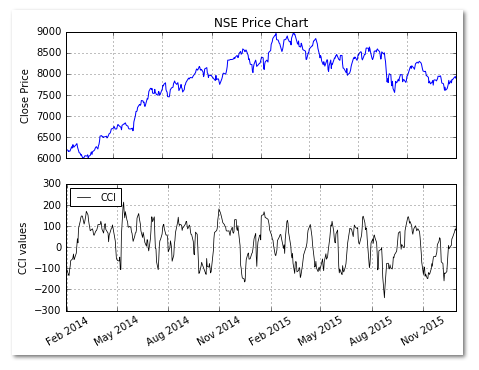Go to the Top

## Ease of Movement (EVM)

Ease of Movement (EVM) is a volume-based oscillator which was developed by Richard Arms. EVM indicates the ease with which the prices rise or fall taking into account the volume of the security. For example, a price rise on a low volume means prices advanced with relative ease, and there was little selling pressure. Positive EVM values imply that the market is moving higher with ease, while negative values indicate an easy decline.

### Estimation

To calculate the EMV we first calculate the distance moved. It is given by:

Distance moved = ((Current High + Current Low)/2 - (Prior High + Prior Low)/2)

We then compute the Box ratio which uses the volume and the high-low range:

Box ratio = (Volume / 100,000,000) / (Current High – Current Low)
EMV = Distance moved / Box ratio

To compute the n-period EMV we take the n-period simple moving average of the 1-period EMV.

### Analysis

Ease of Movement (EMV) can be used to confirm a bullish or a bearish trend. A sustained positive Ease of Movement together with a rising market confirms a bullish trend, while a negative Ease of Movement values with falling prices confirms a bearish trend. Apart from using as a standalone indicator, Ease of Movement (EMV) is also used with other indicators in chart analysis.

### Python code for computing the Ease of Movement (EMV)

Example code: 14-day Ease of Movement (EMV) for AAPL.

In the code below we use the Series, rollingmean, shift, and the join functions to compute the Ease of Movement (EMV) indicator. The Series function is used to form a series which is a one-dimensional array-like object containing an array of data. The rollingmean function takes a time series or a data frame along with the number of periods and computes the mean. The shift function is used to fetch the previous day’s high and low price. The join function joins a given series with a specified series/dataframe.

# Ease Of Movement (EVM) Code

# Load the necessary packages and modules
from pandas_datareader import data as pdr
import matplotlib.pyplot as plt
import yfinance
import pandas as pd

# Ease of Movement
def EVM(data, ndays):
dm = ((data['High'] + data['Low'])/2) - ((data['High'].shift(1) + data['Low'].shift(1))/2)
br = (data['Volume'] / 100000000) / ((data['High'] - data['Low']))
EVM = dm / br
EVM_MA = pd.Series(EVM.rolling(ndays).mean(), name = 'EVM')
data = data.join(EVM_MA)
return data

# Retrieve the AAPL data from Yahoo finance:
data = pdr.get_data_yahoo("AAPL", start="2015-01-01", end="2016-01-01")
data = pd.DataFrame(data)

# Compute the 14-day Ease of Movement for AAPL
n = 14
AAPL_EVM = EVM(data, n)
EVM = AAPL_EVM['EVM']

# Plotting the Price Series chart and the Ease Of Movement below
fig = plt.figure(figsize=(7,5))
ax.set_xticklabels([])
plt.plot(data['Close'],lw=1)
plt.title('AAPL Price Chart')
plt.ylabel('Close Price')
plt.grid(True)
plt.plot(EVM,'k',lw=0.75,linestyle='-',label='EVM(14)')
plt.legend(loc=2,prop={'size':9})
plt.ylabel('EVM values')
plt.grid(True)
plt.setp(plt.gca().get_xticklabels(), rotation=30)


We have also plotted the AAPL Price series and the Ease of Movement (EVM) values below the price chart. We first create an empty figure using the plt.figure function and then create two subplots. The first subplot charts the AAPL price series, while the second subplot charts the EVM values.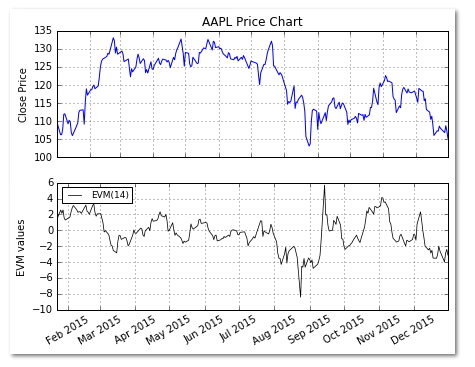Go to the Top

## Moving Average (MA)

The moving average is one of the most widely used technical indicators. It is used along with other technical indicators or it can form the building block for the computation of other technical indicators.

A “moving average” is the average of the asset prices over the “x” number of days/weeks. The term “moving” is used because the group of data moves forward with each new trading day. For each new day, we include the price of that day and exclude the price of the first day in the data sequence.

The most commonly used moving averages are the 5-day, 10-day, 20-day, 50-day, and the 200-day moving averages.

### Estimation

There are different types of moving averages used for analysis, a simple moving average (SMA), weighted moving average (WMA), and the exponential Moving average (EMA).

To compute a 20-day SMA, we take the sum of prices over 20 days and divide it by 20. To arrive at the next data point for the 20-day SMA, we include the price of the next trading day while excluding the price of the first trading day. This way the group of data moves forward.

The SMA assigns equal weights to each price point in the group. When we compute a 20-day WMA, we assign varying weights to each price points. The latest price, i.e. the 20th-day price gets the highest weightage, while the first price gets the lowest weightage. This sum is then divided by the sum of the weights used.

To compute the 20-day EMA, we first compute the very first EMA value using a simple moving average. Then we calculate the multiplier, and thereafter to compute the second EMA value we use the multiplier and the previous day EMA. This formula is used to compute the subsequent EMA values.

SMA: 20 period sum / 20
Multiplier: (2 / (Time periods + 1)) = (2 / (20 + 1)) = 9.52%
EMA: {Close price - EMA(previous day)} x multiplier + EMA(previous day).

### Analysis

The moving average tells whether a trend has begun, ended or reversed. The averaging of the prices produces a smoother line which makes it easier to identify the underlying trend. However, the moving average lags the market action.

A shorter moving average is more sensitive than a longer moving average. However, it is prone to generate false trading signals.

Using a single Moving Average – A single moving average can be used to generate trade signals. When the closing price moves above the moving average, a buy signal is generated and vice versa. When using a single moving average one should select the averaging period in such a way that it is sensitive in generating trading signals and at the same time insensitive in giving out false signals.

Using two Moving Averages – Using a single moving average can be disadvantageous. Hence many traders use two moving averages to generate signals. In this case, a buy signal is generated when the shorter average crosses above the longer average. Similarly, a sell is generated when the shorter crosses below the longer average. Using two moving averages reduces the false signals which are more likely when using a single moving average.

Traders also use three moving averages, like the 5, 10, and 20-day moving average system widely used in the commodity markets.

### Python code for computing Moving Averages for NIFTY

In the code below we use the Series, rolling mean, and the join functions to create the SMA and the EWMA functions. The Series function is used to form a series which is a one-dimensional array-like object containing an array of data. The rolling_mean function takes a time series or a data frame along with the number of periods and computes the mean. The join function joins a given series with a specified series/dataframe.

# Moving Averages Code

# Load the necessary packages and modules
from pandas_datareader import data as pdr
import matplotlib.pyplot as plt
import yfinance
import pandas as pd

# Simple Moving Average
def SMA(data, ndays):
SMA = pd.Series(data['Close'].rolling(ndays).mean(), name = 'SMA')
data = data.join(SMA)
return data

# Exponentially-weighted Moving Average
def EWMA(data, ndays):
EMA = pd.Series(data['Close'].ewm(span = ndays, min_periods = ndays - 1).mean(),
name = 'EWMA_' + str(ndays))
data = data.join(EMA)
return data

# Retrieve the Nifty data from Yahoo finance:
data = pdr.get_data_yahoo("^NSEI", start="2013-01-01", end="2016-01-01")
data = pd.DataFrame(data)
close = data['Close']

# Compute the 50-day SMA for NIFTY
n = 50
SMA_NIFTY = SMA(data,n)
SMA_NIFTY = SMA_NIFTY.dropna()
SMA = SMA_NIFTY['SMA']

# Compute the 200-day EWMA for NIFTY
ew = 200
EWMA_NIFTY = EWMA(data,ew)
EWMA_NIFTY = EWMA_NIFTY.dropna()
EWMA = EWMA_NIFTY['EWMA_200']

# Plotting the NIFTY Price Series chart and Moving Averages below
plt.figure(figsize=(9,5))
plt.plot(data['Close'],lw=1, label='NSE Prices')
plt.plot(SMA,'g',lw=1, label='50-day SMA (green)')
plt.plot(EWMA,'r', lw=1, label='200-day EWMA (red)')
plt.legend(loc=2,prop={'size':11})
plt.grid(True)
plt.setp(plt.gca().get_xticklabels(), rotation=30)


We have also plotted the NIFTY price series, 50-day SMA, and the 200-day EWMA.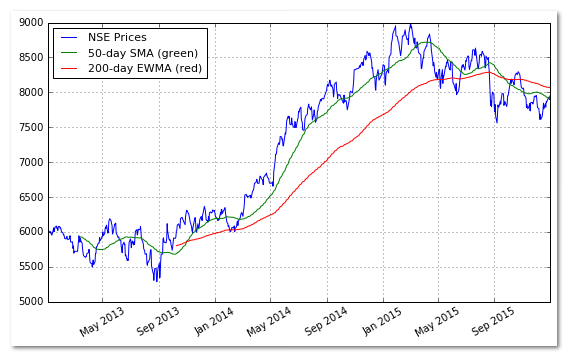Go to the Top

## Rate of Change (ROC)

The Rate of Change (ROC) is a technical indicator that measures the percentage change between the most recent price and the price "n" day’s ago. The indicator fluctuates around the zero line.

If the ROC is rising, it gives a bullish signal, while a falling ROC gives a bearish signal. One can compute ROC based on different periods in order to gauge the short-term momentum or long-term momentum.

### Estimation

ROC = [(Close price today - Close price “n” day’s ago) / Close price “n” day’s ago))]

### Python code for computing Rate of Change (ROC)

Example code: 5-day Rate of Change (ROC) for NIFTY.

In the code below we use the Series, diff, shift, and the join functions to compute the Rate of Change (ROC). The Series function is used to form a series which is a one-dimensional array-like object containing an array of data. The diff function computes the difference in prices between the current day’s price and the price “n” day’s ago. The shift function is used to fetch the previous “n” day’s price. The join function joins a given series with a specified series/dataframe.

# Rate of Change code

# Load the necessary packages and modules
from pandas_datareader import data as pdr
import matplotlib.pyplot as plt
import yfinance
import pandas as pd

# Rate of Change (ROC)
def ROC(data,n):
N = data['Close'].diff(n)
D = data['Close'].shift(n)
ROC = pd.Series(N/D,name='Rate of Change')
data = data.join(ROC)
return data

# Retrieve the NIFTY data from Yahoo finance:
data = pdr.get_data_yahoo("^NSEI", start="2015-06-01", end="2016-01-01")
data = pd.DataFrame(data)

# Compute the 5-period Rate of Change for NIFTY
n = 5
NIFTY_ROC = ROC(data,n)
ROC = NIFTY_ROC['Rate of Change']

# Plotting the Price Series chart and the Ease Of Movement below
fig = plt.figure(figsize=(7,5))
ax.set_xticklabels([])
plt.plot(data['Close'],lw=1)
plt.title('NSE Price Chart')
plt.ylabel('Close Price')
plt.grid(True)
plt.plot(ROC,'k',lw=0.75,linestyle='-',label='ROC')
plt.legend(loc=2,prop={'size':9})
plt.ylabel('ROC values')
plt.grid(True)
plt.setp(plt.gca().get_xticklabels(), rotation=30)


We have also plotted the NIFTY Price series and the Rate of Change (ROC) values below the price chart. We first create an empty figure using the plt.figure function and then create two subplots. The first subplot charts the NIFTY price series, while the second subplot charts the ROC values.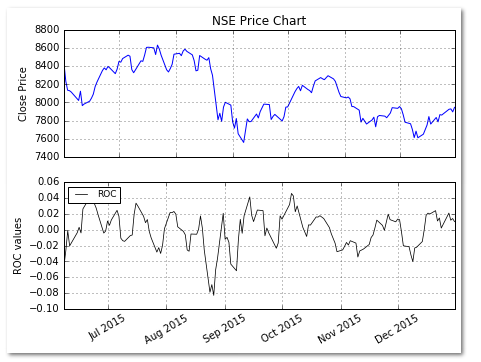Go to the Top

## Bollinger Bands

The concept of Bollinger bands was developed by John Bollinger. These bands comprise of an upper Bollinger band and a lower Bollinger band and are placed two standard deviations above and below a moving average.

Bollinger bands expand and contract based on the volatility. During a period of rising volatility, the bands widen, and they contract as the volatility decreases. Prices are considered to be relatively high when they move above the upper band and relatively low when they go below the lower band.

### Estimation

To create the bands, we first compute the SMA and then use this to compute the bands values.

Middle Band = 20-day simple moving average (SMA)
Upper Band = 20-day SMA + (2 x 20-day standard deviation of price)
Lower Band = 20-day SMA - (2 x 20-day standard deviation of price)

### Analysis

To use Bollinger bands for generating signals, a simple approach would be to use the upper and the lower bands as the price targets. If the price bounces off the lower band and crosses the moving average line, the upper band becomes the upper price target.

In the case of a crossing of the price below the moving average line, the lower band becomes the downside target price.

### Python code for computing Bollinger Bands for NIFTY

In the code below we rolling function to create the Bollinger band function. The mean and the standard deviation methods are used to compute these respective metrics using the close price. Once we have computed the mean and the standard deviation, we compute the upper Bollinger band and the lower Bollinger band. The Bollinger band function is called on the NIFTY price data using the 50-day moving average window.

# Load the necessary packages and modules
from pandas_datareader import data as pdr
import yfinance
import pandas as pd

# Compute the Bollinger Bands
def BBANDS(data, window=n):
MA = data.Close.rolling(window=n).mean()
SD = data.Close.rolling(window=n).std()
data['UpperBB'] = MA + (2 * SD)
data['LowerBB'] = MA - (2 * SD)
return data

# Retrieve the Nifty data from Yahoo finance:
data = pdr.get_data_yahoo("^NSEI", start="2014-01-01", end="2016-01-01")
data = pd.DataFrame(data)

# Compute the Bollinger Bands for NIFTY using the 50-day Moving average
n = 50
NIFTY_BBANDS = BBANDS(data, n)
print(NIFTY_BBANDS)

# Create the plot
pd.concat([NIFTY_BBANDS.Close,NIFTY_BBANDS.UpperBB,NIFTY_BBANDS.LowerBB],axis=1).plot(figsize=(9,5),grid=True)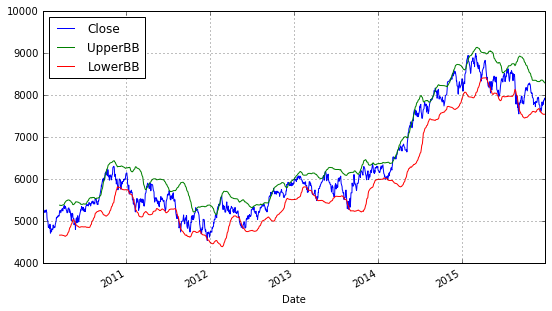Go to the Top

## Force Index

The force index was created by Alexander Elder. The force index takes into account the direction of the stock price, the extent of the stock price movement, and the volume. Using these three elements it forms an oscillator that measures the buying and the selling pressure.

Each of these three factors plays an important role in the determination of the force index. For example, a big advance in prices, which is given by the extent of the price movement, shows a strong buying pressure. A big decline in heavy volume indicates a strong selling pressure.

### Estimation

Example: Computing Force index(1) and Force index(15) period.

The Force index(1) = {Close (current period) - Close (prior period)} x Current period Volume

The Force Index for the 15-day period is an exponential moving average of the 1-period Force Index.

### Analysis

The Force Index can be used to determine or confirm the trend, identify corrections and foreshadow reversals with divergences. A shorter force index is used to determine the short-term trend, while a longer force index, for example, a 100-day force index can be used to determine the long-term trend in prices.

A force index can also be used to identify corrections in a given trend. To do so, it can be used in conjunction with a trend following indicator. For example, one can use a 22-day EMA for trend and a 2-day force index to identify corrections in the trend.

### Python code for computing the Force Index for Apple Inc. (AAPL) Stock

In the code below we use the Series, diff, and the join functions to compute the Force Index. The Series function is used to form a series which is a one-dimensional array-like object containing an array of data. The diff function computes the difference between the current data point and the data point “n” periods/days apart. The join function joins a given series with a specified series/dataframe.

# Load the necessary packages and modules
from pandas_datareader import data as pdr
import matplotlib.pyplot as plt
import yfinance
import pandas as pd

# Force Index
def ForceIndex(data, ndays):
FI = pd.Series(data['Close'].diff(ndays) * data['Volume'], name = 'ForceIndex')
data = data.join(FI)
return data

# Retrieve the Apple Inc. data from Yahoo finance:
data = pdr.get_data_yahoo("AAPL", start="2010-01-01", end="2016-01-01")
data = pd.DataFrame(data)

# Compute the Force Index for AAPL
n = 1
AAPL_ForceIndex = ForceIndex(data,n)
print(AAPL_ForceIndex)


Learn how to use Seaborn Python package to create Heatmaps for data visualisation which can be used for various purposes, including by traders for tracking markets.

If you are seeking more information on Python libraries for Algo Trading, check out our this blog. If you are a coder or a tech professional looking to learn automated trading from experts like Dr. Ernest Chan, Dr, Euan Sinclair,  and industry experts, market practitioners and experienced traders, have a look at our course Executive Programme in Algorithmic Trading (EPAT).

Update: We have noticed that some users are facing challenges while downloading the market data from Yahoo and Google Finance platforms. In case you are looking for an alternative source for market data, you can use Quandl for the same.

Disclaimer: All investments and trading in the stock market involve risk. Any decisions to place trades in the financial markets, including trading in stock or options or other financial instruments is a personal decision that should only be made after thorough research, including a personal risk and financial assessment and the engagement of professional assistance to the extent you believe necessary. The trading strategies or related information mentioned in this article is for informational purposes only.